﻿ 几种世界地图投影的经纬网的数学变换 Mathematical Transformation of Latitude and Longitude Network on Several World Map Projections

Geomatics Science and Technology
Vol. 07  No. 02 ( 2019 ), Article ID: 29912 , 7 pages
10.12677/GST.2019.72017

Mathematical Transformation of Latitude and Longitude Network on Several World Map Projections

Yexun Zhong1,2,3,4, Jiaqi Liu1, Baoqing Hu2,3

1Department of Navigation Engineering, Naval University of Engineering, Wuhan Hubei

2Key Laboratory of Beibu Gulf Environment Change and Resources Use, Ministry of Education, Guangxi Teachers Education University, Nanning Guangxi 3Guangxi Key Laboratory of Earth Surface Processes and Intelligent Simulation (Guangxi Teachers Education University), Nanning Guangxi

4Guangxi Regional Geographical Information Bureau of Surveying and Mapping, Nanning Guangxi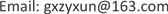Received: Apr. 4th, 2019; accepted: Apr. 19th, 2019; published: Apr. 26th, 2019ABSTRACT

The normal cylindrical projection, pseudo-cylindrical projection, and polyconic projection have different configuration of the longitude and latitude network, but they all belong to the contract graphics. And they all have the characteristics of symmetry transformation with the central meridian λ0 as the axis of symmetry when in the east-west direction, as the equator s0 as the axis of symmetry when in the north-south direction. Also, they all belong to a central symmetrical figure centered on the intersection O of the central meridian and the equator. According to the configuration features and spatial structure of the non-central meridians λi, a convex curved longitude net and a discrete straight longitude net are defined in this paper. According to the configuration characteristics and spatial structure of the non-zero latitudes φj, the inner convex coaxial circular arc latitude net and the equatorial parallel latitude net are defined. On this basis, the mathematical definitions of normal cylindrical projection, pseudo cylindrical projection and polyconic projection are given by the combination of different latitude and longitude nets. According to the rules of set operations, the mathematical transformation between different types of map projections is explained in this paper.

Keywords:Map Projection, Central Meridian, Web of Meridian and Parallel, Reflex Transformation

1海军工程大学导航工程系，湖北 武汉

2南宁师范大学，北部湾环境演变与资源利用省部共建教育部重点实验室，广西 南宁

3南宁师范大学，广西地表过程与智能模拟重点实验室，广西 南宁

4广西测绘地理信息局，广西 南宁1. 引言

2. 合同图形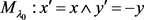(1)

${M}_{{s}_{0}}:{x}^{\prime }=-x\wedge {y}^{\prime }=y$ (2)

3. 用于世界地图的正圆柱投影、伪圆柱投影和多圆锥投影的经纬网

${M}_{{\lambda }_{0}}\in {D}_{\phi \lambda }^{ad}\vee {D}_{\phi \lambda }^{bd}\vee {D}_{\phi \lambda }^{bc}\wedge {M}_{{s}_{0}}\in {D}_{\phi \lambda }^{ad}\vee {D}_{\phi \lambda }^{bd}\vee {D}_{\phi \lambda }^{bc}$ (3)

${M}_{\phi \lambda }\supset {D}_{\phi \lambda }^{ad}\vee {D}_{\phi \lambda }^{bd}\vee {D}_{\phi \lambda }^{bc}$ 的条件下，地图的经纬网具有以中央经线 ${\lambda }_{0}$ 为轴的东西对称：

$\exists {\lambda }_{0}\in {D}_{\phi \lambda }⇒\exists {\lambda }_{i}\notin {\lambda }_{0}\wedge {\lambda }_{{i}^{\prime }}\notin {\lambda }_{0}\wedge {\lambda }_{i}\cap {\phi }_{j}={M}_{ij},{\lambda }_{{i}^{\prime }}\cap {\phi }_{j}={M}_{{i}^{\prime }j}⇒{\lambda }_{0}\perp {M}_{ij}{M}_{{i}^{\prime }j}$ (4)

3.1. 外凸型曲经线族

${D}_{\lambda }^{0}=\left\{{\lambda }_{i}|\forall {\lambda }_{i}\notin {\lambda }_{0}\wedge {\tau }_{i}>0\wedge {T}_{i}>0,i\in {D}_{I}\right\}$ (5)

3.2. 离散型直经线族

${D}_{\lambda }^{*}=\left\{{\lambda }_{i}|\forall {\lambda }_{i}\notin {\lambda }_{0}\wedge {\tau }_{i}=0\wedge {T}_{i}=\infty ,i\in {D}_{I}\right\}$ (6)

3.3. 内凸同轴圆弧型纬线族

${D}_{\phi }^{0}=\left\{{\phi }_{j}|{\phi }_{j}\notin {s}_{0}\wedge {\phi }_{j}\in 2\text{π}{R}_{j},{R}_{j}=g\left({\phi }_{j}\right)={Q}_{j}{M}_{j0},j\in {D}_{J}\right\}$ (7)

(7)式表明，非零纬线为同轴( ${\lambda }_{0}$ )圆弧，圆心为 ${Q}_{j}$ ，半径为 ${R}_{j}$ ，它们是凸部指向赤道的曲线族。

3.4. 赤道平行线型纬线族

${D}_{\phi }^{d}=\left\{{\phi }_{j}^{d}|\forall {\phi }_{j}^{d}\notin {s}_{0}⇒{R}_{j}^{d}=\infty \in {\phi }_{j}^{d},j\in {D}_{J}\right\}$ (8)

(8)式表明，非零纬线均为半径为无穷大的圆弧，也就是直线。于是， ${D}_{\phi }^{d}\cup {s}_{0}={D}_{\phi }$ ，这是赤道平行线型纬线网，又可表达为 ${D}_{\phi }=\left\{{\phi }_{j}^{d}\parallel {s}_{0}|{\phi }_{j}^{d}\notin {s}_{0}\right\}$ ，记作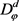4. 正圆柱投影、伪圆柱投影和多圆锥投影的构成和投影变换

4.1. 正圆柱投影

${D}_{\phi \lambda }^{ad}={D}_{\lambda }^{a}\cup {D}_{\phi }^{d}$ (9)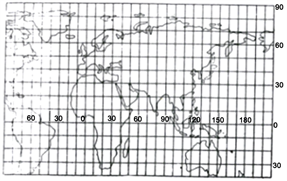Figure 1. Cylindrical projection

4.2. 伪圆柱投影

${D}_{\phi \lambda }^{bd}={D}_{\lambda }^{b}\cup {D}_{\phi }^{d}$ (10)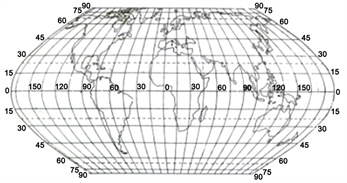Figure 2. Pseudo-cylindrical projection

4.3. 多圆锥投影

${D}_{\phi \lambda }^{bc}={D}_{\lambda }^{b}\cup {D}_{\phi }^{c}$ (11)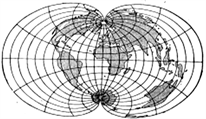Figure 3. Polyconic projection

4.4. 多圆锥投影–伪圆柱投影变换

$\left({D}_{\phi \lambda }^{bc}/{D}_{\phi }^{c}\right)\cup {D}_{\phi }^{d}={D}_{\phi \lambda }^{bd}$ (12)

4.5. 伪圆柱投影–圆柱投影变换

$\left({D}_{\phi \lambda }^{bd}/{D}_{\lambda }^{b}\right)\cup {D}_{\phi }^{a}={D}_{\phi \lambda }^{ad}$ (13)

4.6. 伪圆柱投影–多圆锥投影变换

$\left({D}_{\phi \lambda }^{bd}/{D}_{\phi }^{d}\right)\cup {D}_{\phi }^{c}={D}_{\phi \lambda }^{bc}$ (14)

$\left({D}_{\phi \lambda }^{bd}\cap \varnothing \right)\cup {D}_{\phi \lambda }^{bc}={D}_{\phi \lambda }^{bc}$ (15)

5. 结论

Mathematical Transformation of Latitude and Longitude Network on Several World Map Projections[J]. 测绘科学技术, 2019, 07(02): 117-123. https://doi.org/10.12677/GST.2019.72017

1. 1. 胡毓钜, 龚剑文. 地图投影[M]. 第二版. 北京: 测绘出版社, 1992.

2. 2. 胡毓钜, 龚剑文. 地图投影图集[M]. 第三版. 北京: 测绘出版社, 2006.

3. 3. 钟业勋, 胡宝清. 数理地图学[M]. 第二版. 北京: 测绘出版社, 2017.

4. 4. 钟业勋, 童新华, 李占元. 若干常规地图投影的数学定义[J]. 桂林理工大学学报, 2011, 31(3): 391-394.

5. 5. 吴迪, 钟业勋, 胡宝清, 等. 系列世界地图的经纬网数学模型研究[J]. 海洋测绘, 2018, 38(2): 61-65.

6. 6. 钟业勋, 胡宝清, 童新华, 等. 地图学概念的数学表述研究[M]. 北京: 科学出版社, 2015.

7. 7. 方炳炎. 地图投影计算用表[M]. 北京: 测绘出版社, 1979.

8. 8. 祝国瑞. 地图学[M]. 武汉: 武汉大学出版社, 2004.

9. 9. 吴忠性, 胡毓钜. 地图投影论文集[M]. 北京: 测绘出版社, 1983.

10. 10. 吴忠性, 杨启和. 数学制图学原理[M]. 北京: 测绘出版社, 1989.

11. 11. 张燕顺. 数学的美与理 [M]. 第二版. 北京: 北京大学出版社, 2012.

12. 12. 钟业勋, 边少锋, 胡宝清, 等. 基于不动点子集的常规地图投影分类体系[J]. 广西师范学院学报, 2018, 35(3): 56-60.

13. 13. 汪罗淼, 严虹, 廖义琴. 几何学概论[M]. 北京: 清华大学出版社, 2011.

14. 14. 刘佳奇, 钟业勋, 胡宝清, 等. 基于镜射变换的地图投影新定义体系[J]. 黑龙江工程学院学报, 2017, 31(3): 1-4.

15. 15. 谷超豪. 数学词典[M]. 上海: 上海辞书出版社, 1992.

16. 16. 钟业勋, 胡毓钜, 童新华. 基于不同经纬线类型组合的地图投影新定义[J]. 海洋测绘, 2012, 32(6): 13-16.

17. 17. 楼世博, 孙章, 陈化成. 模糊数学[M]. 北京: 科学出版社, 1985.